# Pipe System Call in C

pipe() is a Linux system function. The pipe() system function is used to open file descriptors, which are used to communicate between different Linux processes. In short, the pipe() function is used for inter-process communication in Linux.  In this article, I am going to show you how to use the pipe() system function in Linux. So, let’s get started.

The syntax of the pipe() function is:

int pipe(int pipefd);

Here, the pipe() function creates a unidirectional data channel for inter-process communication. You pass in an int (Integer) type array pipefd consisting of 2 array element to the function pipe(). Then the pipe() function creates two file descriptors in the pipefd array.

The first element of the pipefd array, pipefd is used for reading data from the pipe.

The second element of the pipefd array, pipefd is used for writing data to the pipe.

On success, the pipe() function returns 0. If an error occurs during pipe initialization, then the pipe() function returns -1.

The pipe() function is defined in the header unistd.h. In order to use the pipe() function in your C program, you must include the header unistd.h as follows:

#include <unistd.h>

For more information on the pipe() system function, check the man page of pipe() with the following command:

\$ man 2 pipe
The man page of pipe().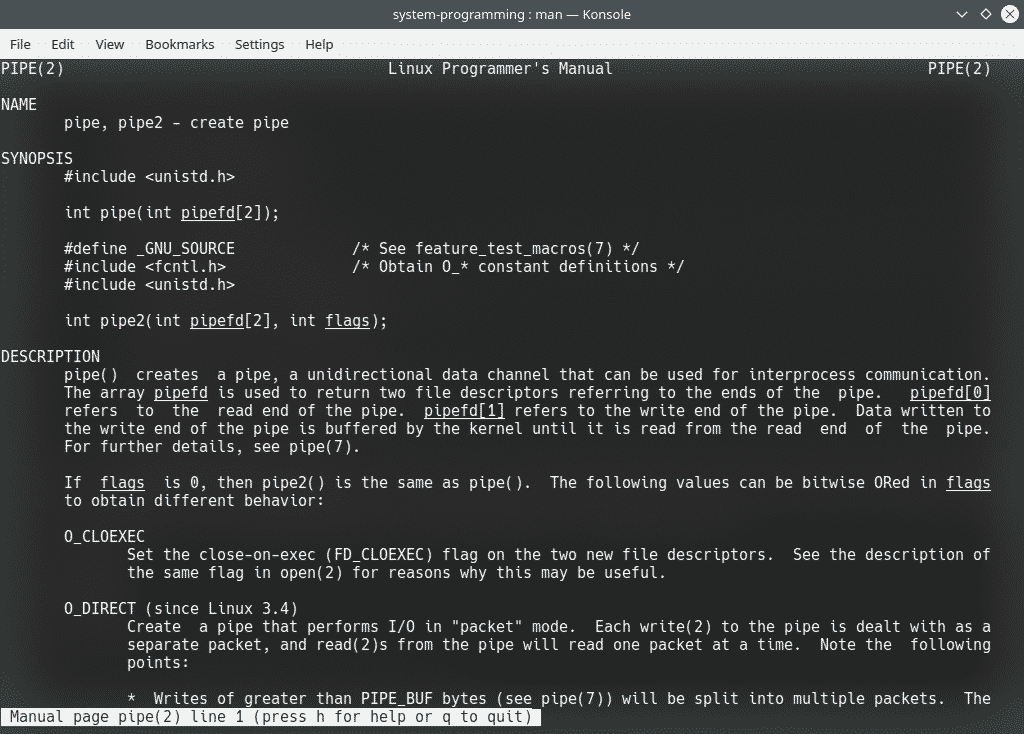### Example 1:

For the first example, create a new C source file 1_pipe.c and type in the following lines of codes.

#include <stdio.h>
#include <stdlib.h>
#include <unistd.h>

int main(void) {
int pipefds;

if(pipe(pipefds) == -1) {
perror("pipe");
exit(EXIT_FAILURE);
}

printf("Read File Descriptor Value: %d\n", pipefds);
printf("Write File Descriptor Value: %d\n", pipefds);

return EXIT_SUCCESS;
}

Here, I included the header file of pipe() unistd.h first with the following line.

#include <unistd.h>

Then, in the main() function, I defined the pipefds two element integer array with the following line.

int pipefds;

Then, I ran the pipe() function to initialize the file descriptors array pipefds as follows.

pipe(pipefds)

I also checked for errors using the return value of the pipe() function. I used the exit() function to terminal the program in case the pipe function fails.

if(pipe(pipefds) == -1) {
perror("pipe");
exit(EXIT_FAILURE);
}

Then, I printed the value of the read and write pipe file descriptors pipefds and pipefds respectively.

printf("Read File Descriptor Value: %d\n", pipefds);
printf("Write File Descriptor Value: %d\n", pipefds);

If you run the program, you should see the following output. As you can see, the value of the read pipe file descriptor pipefds is 3 and write pipe file descriptor pipefds is 4.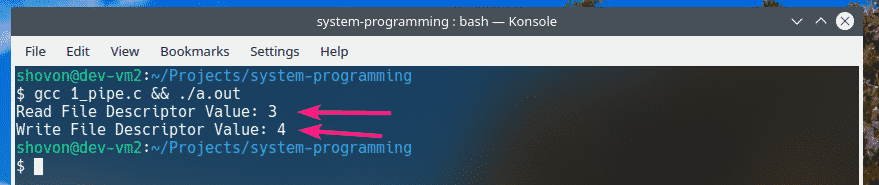### Example 2:

Create another C source file 2_pipe.c and type in the following lines of codes.

#include <stdio.h>
#include <stdlib.h>
#include <unistd.h>
#include <string.h>

int main(void) {
int pipefds;
char buffer;

if(pipe(pipefds) == -1) {
perror("pipe");
exit(EXIT_FAILURE);
}

char *pin = "4128\0";

printf("Writing PIN to pipe...\n");
write(pipefds, pin, 5);
printf("Done.\n\n");

printf("Done.\n\n");

printf("PIN from pipe: %s\n", buffer);

return EXIT_SUCCESS;
}

This program basically shows you how to write to the pipe and read the data you’ve written from the pipe.

Here, I stored a 4-character PIN code into a char array. The length of the array is 5 (including the NULL character \0).

char *pin = "4128\0";

Each ASCII character is 1 byte in size in C. So, to send the 4 digit PIN through the pipe, you must write 5 bytes (4 + 1 NULL character) of data into the pipe.

To write 5 bytes of data (pin) into the pipe, I used the write() function using the write pipe file descriptor pipefds as follows.

write(pipefds, pin, 5);

Now that I have some data in the pipe, I can read it from the pipe using the read() function on the read pipe file descriptor pipefds. As I’ve written 5 bytes of data (pin) into the pipe, I will be reading 5 bytes of data from the pipe as well. The data read will be stored in the buffer character array. As I will be reading 5 bytes of data from the pipe, the buffer character array must be at least 5 bytes long.

I have defined the buffer character array in the beginning of the main() function.

char buffer;

Now, I can read the PIN from the pipe and store it in the buffer array with the following line.

Now that I have read the PIN from the pipe, I can print it using the printf() function as usual.

printf("PIN from pipe: %s\n", buffer);

Once I run the program, the correct output is displayed as you can see.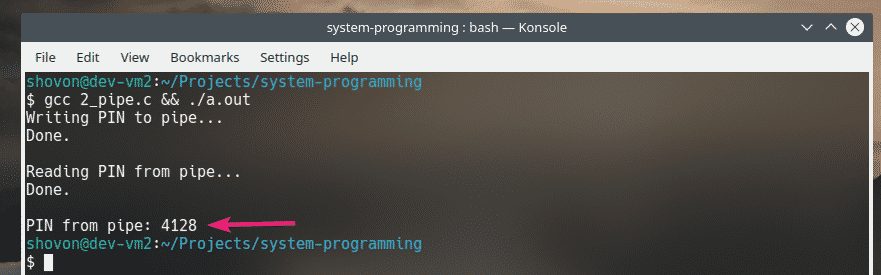### Example 3:

Create a new C source file 3_pipe.c as type in the following lines of codes.

#include <stdio.h>
#include <stdlib.h>
#include <unistd.h>
#include <sys/types.h>
#include <sys/wait.h>
int main(void) {
int pipefds;
char *pin;
char buffer;

if(pipe(pipefds) == -1) {
perror("pipe");
exit(EXIT_FAILURE);
}

pid_t pid = fork();

if(pid == 0) { // in child process
pin = "4821\0"; // PIN to send
write(pipefds, pin, 5); // write PIN to pipe

printf("Generating PIN in child and sending to parent...\n");
sleep(2); // intentional delay
exit(EXIT_SUCCESS);
}

if(pid > 0) { // in main process
wait(NULL); // wait for child process to finish
close(pipefds); // close write fd

}

return EXIT_SUCCESS;
}

In this example, I showed you how to use pipe for inter-process communication. I’ve sent a PIN from the child process to the parent process using a pipe. Then read the PIN from the pipe in the parent process and printed it from the parent process.

First, I’ve created a child process using fork() function.

pid_t pid = fork();

Then, in the child process (pid == 0), I wrote the PIN to the pipe using the write() function.

write(pipefds, pin, 5);

Once the PIN is written to the pipe from the child process, the parent process (pid > 0) read it from the pipe using the read() function.

Then, the parent process printed the PIN using printf() function as usual.

As you can see, running the program gives the expected result.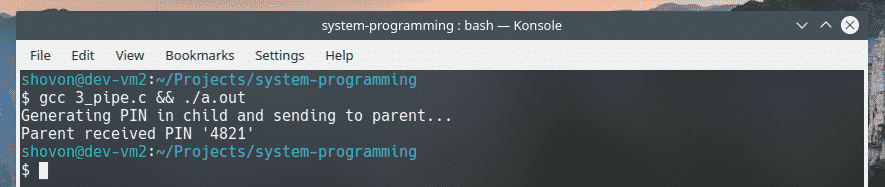### Example 4:

Create a new C source file 4_pipe.c as type in the following lines of codes.

#include <stdio.h>
#include <stdlib.h>
#include <unistd.h>
#include <sys/types.h>
#include <sys/wait.h>

#define PIN_LENGTH 4
#define PIN_WAIT_INTERVAL 2

void getPIN(char pin[PIN_LENGTH + 1]) {
srand(getpid() + getppid());

pin = 49 + rand() % 7;

for(int i = 1; i < PIN_LENGTH; i++) {
pin[i] = 48 + rand() % 7;
}

pin[PIN_LENGTH] = '\0';
}

int main(void) {
while(1) {
int pipefds;
char pin[PIN_LENGTH + 1];
char buffer[PIN_LENGTH + 1];

pipe(pipefds);

pid_t pid = fork();

if(pid == 0) {
getPIN(pin); // generate PIN
write(pipefds, pin, PIN_LENGTH + 1); // write PIN to pipe

printf("Generating PIN in child and sending to parent...\n");

sleep(PIN_WAIT_INTERVAL); // delaying PIN generation intentionally.

exit(EXIT_SUCCESS);
}

if(pid > 0) {
wait(NULL); // waiting for child to finish

close(pipefds); // close write fd
printf("Parent received PIN '%s' from child.\n\n", buffer);
}
}

return EXIT_SUCCESS;
}

This example is the same as Example 3. The only difference is that this program continuously creates a child process, generates a PIN in the child process and sends the PIN to the parent process using a pipe.

The parent process then reads the PIN from the pipe and prints it.

This program generates a new PIN_LENGTH PIN every PIN_WAIT_INTERVAL seconds.

As you can see, the program works as expected.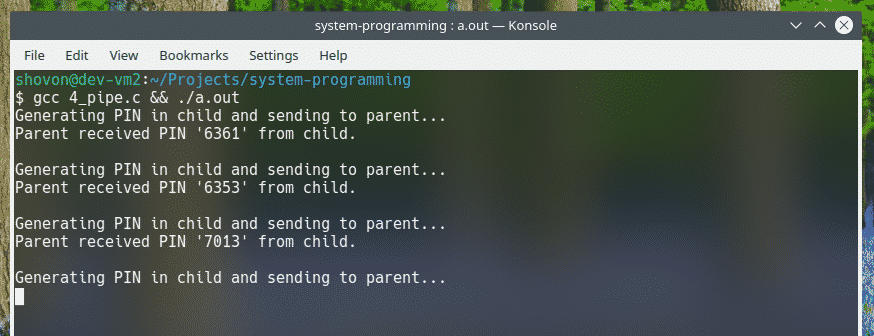You can only stop the program by pressing <Ctrl> + C.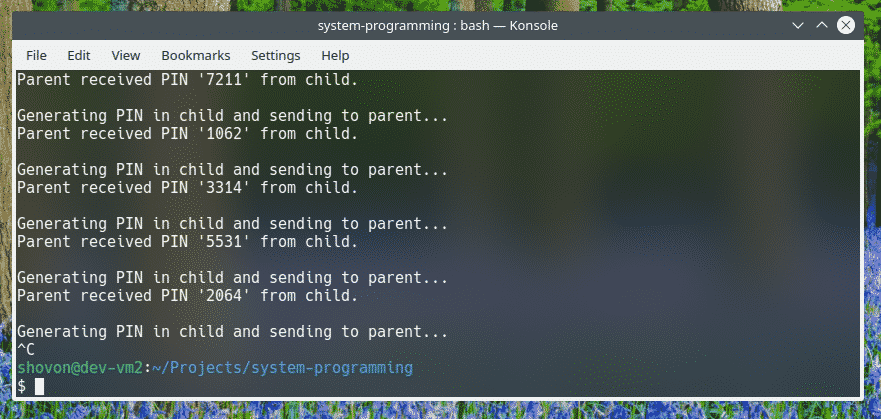So, this is how you use the pipe() system call in C programming language. Thanks for reading this article.